# 陈数Chern number的计算（Kubo公式，附Python代码）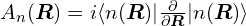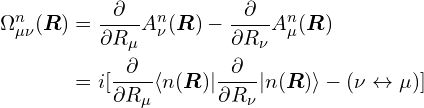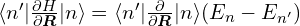for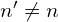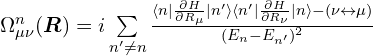"""
This code is supported by the website: https://www.guanjihuan.com
The newest version of this code is on the web page: https://www.guanjihuan.com/archives/16148
"""

import numpy as np
from math import *
import time

def hamiltonian(kx, ky):  # one QAH model with Chern number = 2
t1 = 1.0
t2 = 1.0
t3 = 0.5
m = -1.0
matrix = np.zeros((2, 2), dtype=complex)
matrix[0, 1] = 2*t1*cos(kx)-1j*2*t1*cos(ky)
matrix[1, 0] = 2*t1*cos(kx)+1j*2*t1*cos(ky)
matrix[0, 0] = m+2*t3*sin(kx)+2*t3*sin(ky)+2*t2*cos(kx+ky)
matrix[1, 1] = -(m+2*t3*sin(kx)+2*t3*sin(ky)+2*t2*cos(kx+ky))
return matrix

def main():
start_time = time.time()
n = 200       # integration
delta = 1e-6  # derivation
chern_number = 0
for kx in np.arange(-pi, pi, 2*pi/n):
for ky in np.arange(-pi, pi,2*pi/n):
H = hamiltonian(kx, ky)
eigenvalue, eigenvector = np.linalg.eig(H)
vector_0 = eigenvector[:, np.argsort(np.real(eigenvalue))]
vector_1 = eigenvector[:, np.argsort(np.real(eigenvalue))]
eigenvalue = np.sort(np.real(eigenvalue))

H_delta_kx = hamiltonian(kx+delta, ky)-hamiltonian(kx, ky)
H_delta_ky = hamiltonian(kx, ky+delta)-hamiltonian(kx, ky)

berry_curvature = 1j*(np.dot(np.dot(np.dot(np.dot(np.dot(vector_0.transpose().conj(), H_delta_kx/delta), vector_1), vector_1.transpose().conj()), H_delta_ky/delta), vector_0)- np.dot(np.dot(np.dot(np.dot(np.dot(vector_0.transpose().conj(), H_delta_ky/delta), vector_1), vector_1.transpose().conj()), H_delta_kx/delta), vector_0))/(eigenvalue-eigenvalue)**2

chern_number = chern_number + berry_curvature*(2*pi/n)**2
chern_number = chern_number/(2*pi)
print('Chern number = ', chern_number)
end_time = time.time()
print('运行时间(min)=', (end_time-start_time)/60)

if __name__ == '__main__':
main()

5,246 次浏览

【说明：本站主要是个人的一些笔记和代码分享，内容可能会不定期修改。为了使全网显示的始终是最新版本，这里的文章未经同意请勿转载。引用请注明出处：https://www.guanjihuan.com

## 26 thoughts on “陈数Chern number的计算（Kubo公式，附Python代码）”

1.匿名说道：

您好!请问我在其他晶格中，使用该方法时，假如是蜂窝晶格，其布里渊区的选取可以平移成方形来进行计算吗，感谢您的回复。

1.guanjihuan说道：

应该是可以的，相邻的布里渊区具有等价性。

2.匿名说道：

您好，我使用当前的代码，对角化函数改为eigh（对角化厄米矩阵）而非eig，最后陈数就不对，是什么原因呢？

1.guanjihuan说道：

结果一样的呀

1.匿名说道：

找到问题了，因为arm版本的mac下numpy的数值库有bug.....多谢博主啦！

3.匿名说道：

请问kubo公式在计算简并能带的陈数是要怎么处理？En-En’=0，最后会有NAN的出现。

1.guanjihuan说道：
4.匿名说道：

用matlab计算，计算精度完全不一样。
用上面网友给处的方法，算出来的结果是2.0066-7.6367e-20i
自己用matlab写wilson loop方法也是。
精度只能到小数点后2~3位。
想算得足够小， n = 200 # integration 项要非常大。
不知道是什么原因导致的

1.匿名说道：

哦我找到问题了，是重复计算了边界
matlab中生成数组 kx=-pi:L:pi;
结果是 [-pi, -pi+L,-pi+2L,....,pi-L,pi]
这里出现了-pi和pi, 而这两个点是等价的。

这个点重复统计了两次，
以上面网友给出的matlab程序为例：
for循环应该去掉最后一位，改成
for ii=1:length(kx0)-1
for jj=1:length(ky0)-1
.....
end
end

1.guanjihuan说道：

嗯，Matlab的取值范围是左闭右闭的。

5.匿名说道：

写了个matlab版的，结果对的上：

%kubo公式，特点是不用加波函数规范
function []=main_kubo_chen()
tic
n=200;%布里渊区积分离散参数
delta=1e-6;%求导步长,应该是要比积分步长小
c_num=0;%陈数初始化
band_index=1;%算哪条陈数的能带，本征值从低到高
N=2;%哈密顿量矩阵的阶数，也是波函数个数，根据实际情况写
kx0=-pi:2*pi/n:pi;
ky0=kx0;%在第一布里渊区积分

for ii=1:length(kx0)
for jj=1:length(ky0)
kx=kx0(ii);
ky=ky0(jj);
H=hami(kx,ky);
[eigen_vecor,eigen_value]=eig(H);

[e_value,index]=sort(real(diag(eigen_value)));
wave_fun_matrix=eigen_vecor(:,index);%按照本征值从小到大排列波函数

D_H_kx = (hami(kx+delta, ky)-hami(kx, ky))/delta;%矩阵，求导
D_H_ky = (hami(kx, ky+delta)-hami(kx, ky))/delta;%矩阵

berry_cur=0;%贝利曲率初始化

wave_fun0=wave_fun_matrix(:,band_index);%|n>
value0=e_value(band_index);%En
for uu=1:N
if uu==band_index
continue
end

temp_wave_fun=wave_fun_matrix(:,uu);%|n'>
temp_value=e_value(uu);%En'

a1=wave_fun0'*D_H_kx*temp_wave_fun*temp_wave_fun'*D_H_ky*wave_fun0;
a2=wave_fun0'*D_H_ky*temp_wave_fun*temp_wave_fun'*D_H_kx*wave_fun0;

berry_cur=berry_cur+1j*(a1-a2)/(value0-temp_value)^(2);
end
%贝利曲率计算完毕,berry_cur
c_num=c_num+berry_cur*((2*pi/n)^2);
end
end

c_num=c_num/(2*pi);

disp(['第',num2str(band_index),'条能带陈数为:',num2str(c_num)])
toc
end

function  [out]=hami(kx,ky)
%一个测试哈密顿量
t1 = 1.0;
t2 = 1.0;
t3 = 0.5;
m = -1.0;
matrix =zeros(2);
matrix(1, 2) = 2*t1*cos(kx)-1j*2*t1*cos(ky);
matrix(2,1) = 2*t1*cos(kx)+1j*2*t1*cos(ky);
matrix(1,1) = m+2*t3*sin(kx)+2*t3*sin(ky)+2*t2*cos(kx+ky);
matrix(2,2) = -(m+2*t3*sin(kx)+2*t3*sin(ky)+2*t2*cos(kx+ky));
out=matrix;
end

1.guanjihuan说道：

嗯，用的公式一样的吧

1.匿名说道：

就是根据您py版改写的

1.匿名说道：

这个久保公式法在有能带兼并的时候咋办，En-En’=0，发散了，数值是缺失值NAN

1.guanjihuan说道：

我可以先试着加微扰打开带隙吧。或者把价带波函数当成整体来算？这个我还没验证过。

1.匿名说道：
1.guanjihuan说道：

感谢提供的文献！

2.匿名说道：

你好，这个matlab代码可以画3维的贝里曲率图吗？z轴是贝里曲率，x、y轴分别是kx、ky那种，谢谢~

1.guanjihuan说道：

可以的，不求和就是贝里曲率的分布，求和就是陈数。

1.匿名说道：

可否具体出一个画3维贝里曲率图形的matlab代码，我修改了一番这个评论区的求陈数的代码，没有画出来贝里曲率图，感谢指导~

1.guanjihuan说道：

其实也不需要改多少，最后没有求和，就是贝里曲率分布。目前没有matlab代码，可以参考这篇：贝里曲率的计算（附Python代码）

1.匿名说道：

好嘞，谢谢~

6.匿名说道：

算的是最低能带对应的陈数吗

1.guanjihuan说道：

嗯，是价带，这里是两条带的最低带。

7.匿名说道：

好像计算陈数还有个kubo公式的方法，威尔逊环等方法，我见过的就有四五种。

1.guanjihuan说道：

嗯，是还有其他几种方法，都是等价的。